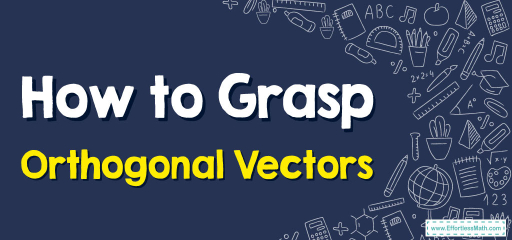# How to Grasp Orthogonal Vectors

Orthogonal vectors are vectors that meet at a right angle, meaning they are perpendicular to each other. In the context of vectors, "orthogonal" is essentially a sophisticated way of saying "perpendicular".## Step-by-step Guide to Understand Orthogonal Vectors

Here is a step-by-step guide to understanding orthogonal vectors:

### Step 1: Prelude – The Enchanted Realm of Vectors

Before we embark on our voyage into orthogonality, we must first delve into the world of vectors: magnificent entities with both magnitude and direction. Think of them as arrows pointing the way through a multidimensional space.

### Step 2: Orthogonality Unveiled – The Heart of Perpendicularity

At its essence, orthogonality is the romance of perpendicularity transposed into the domain of vectors.

1. The Geometric Overture:
• Two vectors are termed orthogonal if they meet at a pristine right angle in space. This encapsulates the notion of perpendicularity but with a touch of algebraic finesse.
2. The Algebraic Dance:
• When vectors are orthogonal, their dot product is zero. This is the algebraic hallmark of orthogonality.
• Formally, for two vectors $$\overrightarrow{A}$$ and $$\overrightarrow{B}$$, if: $$\overrightarrow{A}⋅\overrightarrow{B}=0$$, then the vectors are orthogonal.

### Step 3: The Dot Product – The Key to Orthogonality’s Secret Garden

The dot product of two vectors sometimes termed the scalar product, is a scalar (number) that provides insight into the angle between vectors.

1. Recapitulation of Dot Product:
• For vectors $$\overrightarrow{A}=A_x​i+A_y​j$$​ and $$\overrightarrow{B}=B_x​i+B_y​j$$​, $$\overrightarrow{A}⋅\overrightarrow{B}=A_x​B_x​+A_y​B_y$$​.
2. Unlocking Orthogonality:
• If $$\overrightarrow{A}⋅\overrightarrow{B}=0$$, it unveils the vectors’ orthogonal nature.

### Step 4: Traversing Orthogonal Dimensions:

1. $$2D$$ Orthogonal Ballet:
• In a $$2D$$ space, orthogonal vectors seem to dance a duet, one on the $$x$$-axis and the other on the $$y$$-axis, meeting at a crisp $$90$$-degree angle.
2. $$3D$$ Orthogonal Symphony:
• In the vast expanse of $$3D$$ space, vectors can be orthogonal in a myriad of configurations. One might soar along the $$z$$-axis while the other glides along the $$x$$-axis, yet they never truly ‘touch’ except in the perpendicular sense.

### Step 5: Applications – Where Orthogonality Reigns Supreme

1. Linear Algebra: Orthogonal vectors are the bedrock of orthogonal matrices, aiding in efficient computations and transformations.
2. Signal Processing: Orthogonality prevents interference between signals, ensuring clarity and precision.
3. Computer Graphics: They help in defining normals to surfaces, facilitating realistic rendering of light and shadow.

### Step 6: Concluding Reflections – The Awe of Right Angles

In the vast universe of vectors, orthogonality stands as a beacon of structured order, a testament to the beauty of right angles in a world of direction and magnitude. Whether you’re an artist captivated by perpendicular lines or a mathematician mesmerized by algebraic properties, the concept of orthogonal vectors offers a harmonious blend of geometry and algebra. Embrace the elegance, and let the orthogonal vectors illuminate your mathematical pursuits!

### Examples:

Example 1:

Given vectors: $$\overrightarrow{R}=(5,7)$$, and $$\overrightarrow{S}=(7,−5)$$, determine if the vectors are orthogonal

Solution:

To determine if the vectors are orthogonal:

Compute their dot product: $$\overrightarrow{R}⋅\overrightarrow{S}=5×7+7×(−5) =35−35 =0$$

Since their dot product is zero, vectors $$\overrightarrow{R}$$ and $$\overrightarrow{S}$$ are orthogonal.

Example 2:

Given vectors: $$\overrightarrow{T}=(1,−3)$$, and $$\overrightarrow{U}=(3,1)$$, determine if the vectors are orthogonal

Solution:

Again, compute their dot product: $$\overrightarrow{T}⋅\overrightarrow{U}=1×3+(−3)×1=3−3=0$$

Once again, since their dot product is zero, vectors $$\overrightarrow{T}$$ and $$\overrightarrow{U}$$ are orthogonal.

### What people say about "How to Grasp Orthogonal Vectors - Effortless Math: We Help Students Learn to LOVE Mathematics"?

No one replied yet.

X
30% OFF

Limited time only!

Save Over 30%

SAVE $5 It was$16.99 now it is \$11.99